# Scatter Plots

library("lessR")
style()
## theme set to "colors"

To illustrate, first read the Employee data included as part of lessR.

d <- Read("Employee")
##
## >>> Suggestions
## Details about your data, Enter:  details()  for d, or  details(name)
##
## Data Types
## ------------------------------------------------------------
## character: Non-numeric data values
## integer: Numeric data values, integers only
## double: Numeric data values with decimal digits
## ------------------------------------------------------------
##
##     Variable                  Missing  Unique
##         Name     Type  Values  Values  Values   First and last values
## ------------------------------------------------------------------------------------------
##  1     Years   integer     36       1      16   7  NA  15 ... 1  2  10
##  2    Gender character     37       0       2   M  M  M ... F  F  M
##  3      Dept character     36       1       5   ADMN  SALE  SALE ... MKTG  SALE  FINC
##  4    Salary    double     37       0      37   53788.26  94494.58 ... 56508.32  57562.36
##  5    JobSat character     35       2       3   med  low  low ... high  low  high
##  6      Plan   integer     37       0       3   1  1  3 ... 2  2  1
##  7       Pre   integer     37       0      27   82  62  96 ... 83  59  80
##  8      Post   integer     37       0      22   92  74  97 ... 90  71  87
## ------------------------------------------------------------------------------------------

lessR provides many versions of a scatter plot with its Plot() function.

## Two Variables

The regular scatterplot of two continuous variables.

Plot(Years, Salary)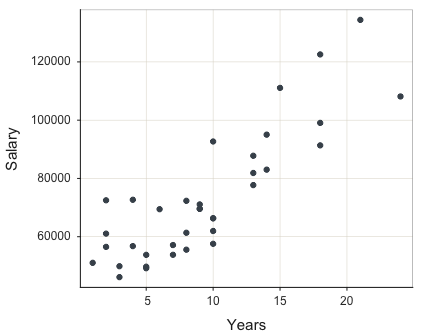## >>> Suggestions
## Plot(Years, Salary, fit="lm", fit_se=c(.90,.99))  # fit line, standard errors
## Plot(Years, Salary, out_cut=.10)  # label top 10% potential outliers
## Plot(Years, Salary, enhance=TRUE)  # many options
##
##
## >>> Pearson's product-moment correlation
##
## Number of paired values with neither missing, n = 36
##
##
## Sample Correlation of Years and Salary: r = 0.852
##
##
## Hypothesis Test of 0 Correlation:  t = 9.501,  df = 34,  p-value = 0.000
## 95% Confidence Interval for Correlation:  0.727 to 0.923

The enhanced scatterplot with parameter enhance.

Plot(Years, Salary, enhance=TRUE)
## [Ellipse with Murdoch and Chow's function ellipse from the ellipse package]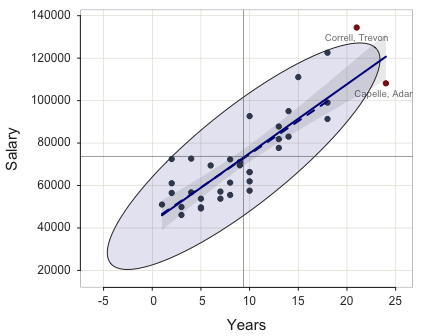## >>> Suggestions
## Plot(Years, Salary, fit="lm", fit_se=c(.90,.99))  # fit line, standard errors
## Plot(Years, Salary, out_cut=.10)  # label top 10% potential outliers
##
##
## >>> Pearson's product-moment correlation
##
## Number of paired values with neither missing, n = 36
##
##
## Sample Correlation of Years and Salary: r = 0.852
##
##
## Hypothesis Test of 0 Correlation:  t = 9.501,  df = 34,  p-value = 0.000
## 95% Confidence Interval for Correlation:  0.727 to 0.923
## >>> Outlier analysis with Mahalanobis Distance
##
##   MD                  ID
## -----               -----
## 8.14     Correll, Trevon
## 7.84       Capelle, Adam
##
## 5.63  Korhalkar, Jessica
## 5.58       James, Leslie
## 3.75         Hoang, Binh
## ...                 ...

Plot the scatterplot with the non-linear best fit "loess" line. The three available values for the fit line are "loess" for non-linear, "lm" for linear, and "null" for the null model line, the flat line at the mean of $$y$$. Also, setting fit to TRUE sets fit to"loess".

For emphasis set plot_errors to TRUE to plot the residuals from the line.

Plot(Years, Salary, fit="loess", plot_errors=TRUE)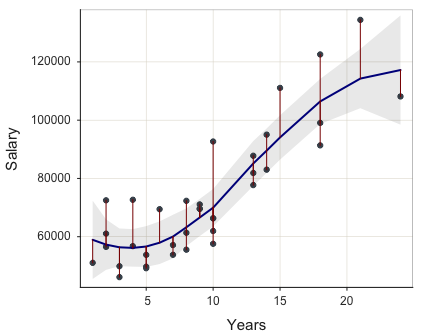## >>> Suggestions
## Plot(Years, Salary, out_cut=.10)  # label top 10% potential outliers
## Plot(Years, Salary, enhance=TRUE)  # many options
##
##
## >>> Pearson's product-moment correlation
##
## Number of paired values with neither missing, n = 36
##
##
## Sample Correlation of Years and Salary: r = 0.852
##
##
## Hypothesis Test of 0 Correlation:  t = 9.501,  df = 34,  p-value = 0.000
## 95% Confidence Interval for Correlation:  0.727 to 0.923

Map variable Pre to the points with the size parameter, a bubble plot.

Plot(Years, Salary, size=Pre)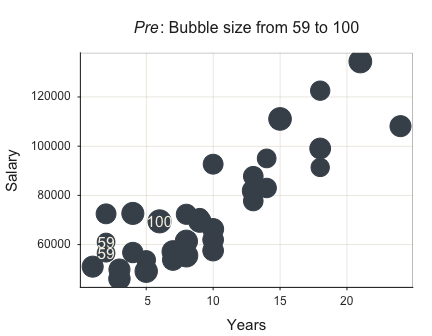## >>> Suggestions
## Plot(Years, Salary, fit="lm", fit_se=c(.90,.99))  # fit line, standard errors
## Plot(Years, Salary, out_cut=.10)  # label top 10% potential outliers
## Plot(Years, Salary, enhance=TRUE)  # many options
##
##
## >>> Pearson's product-moment correlation
##
## Number of paired values with neither missing, n = 36
##
##
## Sample Correlation of Years and Salary: r = 0.852
##
##
## Hypothesis Test of 0 Correlation:  t = 9.501,  df = 34,  p-value = 0.000
## 95% Confidence Interval for Correlation:  0.727 to 0.923

Plot against levels of categorical variable Gender with the by parameter.

Plot(Years, Salary, by=Gender)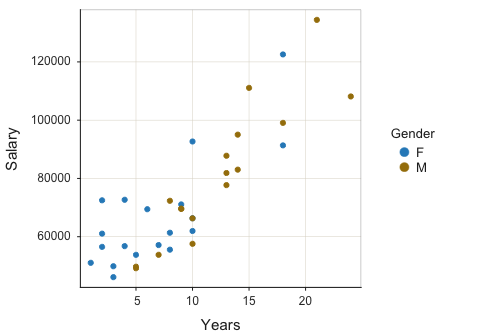## >>> Suggestions
## Plot(Years, Salary, fit="lm", fit_se=c(.90,.99))  # fit line, standard errors
## Plot(Years, Salary, out_cut=.10)  # label top 10% potential outliers
## Plot(Years, Salary, enhance=TRUE)  # many options
##
##
## >>> Pearson's product-moment correlation
##
## Number of paired values with neither missing, n = 36
##
##
## Sample Correlation of Years and Salary: r = 0.852
##
##
## Hypothesis Test of 0 Correlation:  t = 9.501,  df = 34,  p-value = 0.000
## 95% Confidence Interval for Correlation:  0.727 to 0.923

The categorical variable can also generate Trellis plots with the by parameter.

Plot(Years, Salary, by1=Gender)
## [Trellis graphics from Deepayan Sarkar's lattice package]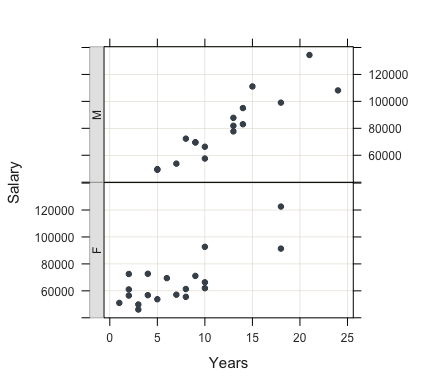Two categorical variables result in a bubble plot of their joint frequencies.

Plot(Dept, Gender)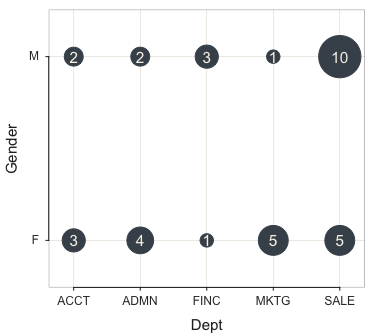## >>> Suggestions
## Plot(Dept, Gender, size_cut=FALSE)
## Plot(Dept, Gender, trans=.8, bg="off", grid="off")
## SummaryStats(Dept, Gender)  # or ss
##
##
## Joint and Marginal Frequencies
## ------------------------------
##
##        Dept
## Gender   ACCT ADMN FINC MKTG SALE Sum
##   F         3    4    1    5    5  18
##   M         2    2    3    1   10  18
##   Sum       5    6    4    6   15  36
##
##
## Cramer's V: 0.415
##
## Chi-square Test:  Chisq = 6.200, df = 4, p-value = 0.185
## >>> Low cell expected frequencies, chi-squared approximation may not be accurate

## Distribution of a Single Variable

The default plot for a single continuous variable includes not only the scatterplot, but also the violin plot and box plot, with outliers identified. Call this plot the VBS plot.

Plot(Salary)
## [Violin/Box/Scatterplot graphics from Deepayan Sarkar's lattice package]
## >>> Suggestions
## Plot(Salary, out_cut=2, fences=TRUE, vbs_mean=TRUE)  # Label two outliers ...
## Plot(Salary, box_adj=TRUE)  # Adjust boxplot whiskers for asymmetry
##
## --- Salary ---
## Present: 37
## Missing: 0
## Total  : 37
##
## Mean         : 73795.557
## Stnd Dev     : 21799.533
## IQR          : 31012.560
## Skew         : 0.190   [medcouple, -1 to 1]
##
## Minimum      : 46124.970
## Lower Whisker: 46124.970
## 1st Quartile : 56772.950
## Median       : 69547.600
## 3rd Quartile : 87785.510
## Upper Whisker: 122563.380
## Maximum      : 134419.230
##
##
## (Box plot) Outliers: 1
##
## Small      Large
## -----      -----
##            Correll, Trevon 134419.23
##
##
## Number of duplicated values: 0
##
##
## Parameter values (can be manually set)
## -------------------------------------------------------
## size: 0.61      size of plotted points
## jitter_y: 0.45  random vertical movement of points
## jitter_x: 0.00  random horizontal movement of points
## bw: 9529.04     set bandwidth higher for smoother edges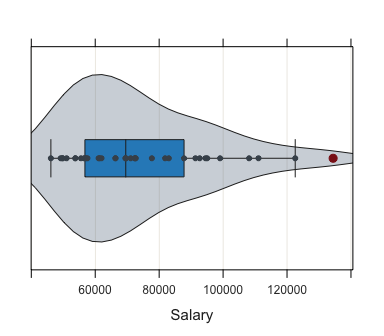For a single categorical variable, get the corresponding bubble plot of frequencies.

Plot(Dept)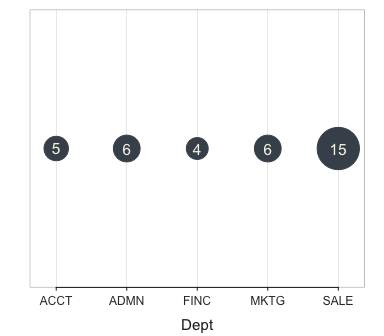## >>> Suggestions
## Plot(Dept, color_low="lemonchiffon2", color_hi="maroon3")
## Plot(Dept, values="count")  # scatter plot of counts
##
##
## --- Dept ---
##
##
##                 ACCT   ADMN   FINC   MKTG   SALE    Total
## Frequencies:       5      6      4      6     15       36
## Proportions:   0.139  0.167  0.111  0.167  0.417    1.000
##
##
## Chi-squared test of null hypothesis of equal probabilities
##   Chisq = 10.944, df = 4, p-value = 0.027

## Cleveland Dot Plot

The Cleveland dot plot, here for a single variable, has row names on the y-axis. The default plots sorts by the value plotted.

Plot(Salary, row_names)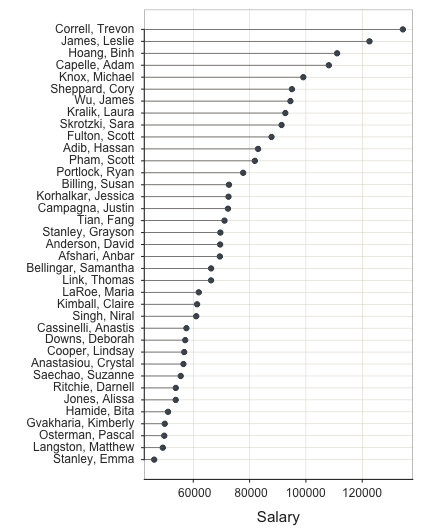## >>> Suggestions
## Plot(Salary, y=row_names, sort_yx=FALSE, segments_y=FALSE)
##
##
##
## --- Salary ---
##
##      n   miss      mean        sd       min       mdn       max
##      37      0   73795.6   21799.5   46125.0   69547.6  134419.2
##
##
## (Box plot) Outliers: 1
##
## Small      Large
## -----      -----
##             134419.2

The standard scatterplot version of a Cleveland dot plot.

Plot(Salary, row_names, sort_yx="0", segments_y=FALSE)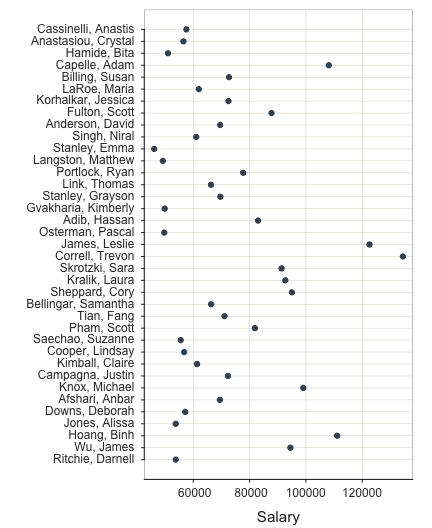## >>> Suggestions
##
##
##
## --- Salary ---
##
##      n   miss      mean        sd       min       mdn       max
##      37      0   73795.6   21799.5   46125.0   69547.6  134419.2
##
##
## (Box plot) Outliers: 1
##
## Small      Large
## -----      -----
##             134419.2

This Cleveland dot plot has two x-variables, indicated as a standard R vector with the c() function. In this situation the two points on each row are connected with a line segment. By default the rows are sorted by distance between the successive points.

Plot(c(Pre, Post), row_names)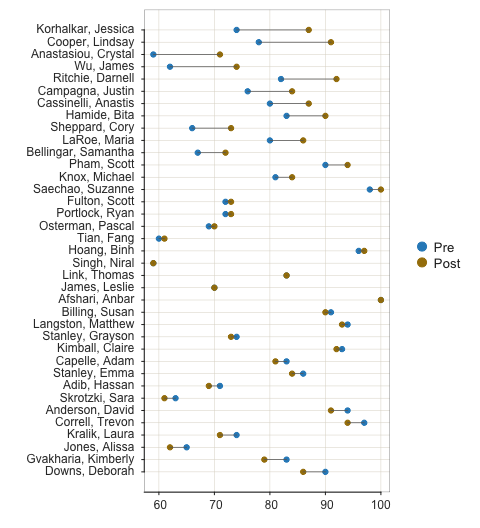## >>> Suggestions
## Plot(c(Pre, Post), y=row_names, sort_yx=FALSE, segments_y=FALSE)
##
##
##
## --- Pre ---
##
##      n   miss    mean      sd     min     mdn     max
##      37      0    78.8    12.0    59.0    80.0   100.0
##
##
## --- Post ---
##
##      n   miss    mean      sd     min     mdn     max
##      37      0    81.0    11.6    59.0    84.0   100.0
##
##
## No (Box plot) outliers
##
##
##  n  diff  Row
## ---------------------------
##  1 13.0 Korhalkar, Jessica
##  2 13.0 Cooper, Lindsay
##  3 12.0 Anastasiou, Crystal
##  4 12.0 Wu, James
##  5 10.0 Ritchie, Darnell
##  6  8.0 Campagna, Justin
##  7  7.0 Cassinelli, Anastis
##  8  7.0 Hamide, Bita
##  9  7.0 Sheppard, Cory
## 10  6.0 LaRoe, Maria
## 27 -1.0 Kimball, Claire
## 28 -2.0 Capelle, Adam
## 29 -2.0 Stanley, Emma
## 30 -2.0 Adib, Hassan
## 31 -2.0 Skrotzki, Sara
## 32 -3.0 Anderson, David
## 33 -3.0 Correll, Trevon
## 34 -3.0 Kralik, Laura
## 35 -3.0 Jones, Alissa
## 36 -4.0 Gvakharia, Kimberly
## 37 -4.0 Downs, Deborah

## Time Series

Read time series data of stock Price for three companies: Apple, IBM, and Intel. The data table is in long form, part of lessR.

d <- Read("StockPrice")
##
## >>> Suggestions
## Details about your data, Enter:  details()  for d, or  details(name)
##
## Data Types
## ------------------------------------------------------------
## character: Non-numeric data values
## Date: Date with year, month and day
## double: Numeric data values with decimal digits
## ------------------------------------------------------------
##
##     Variable                  Missing  Unique
##         Name     Type  Values  Values  Values   First and last values
## ------------------------------------------------------------------------------------------
##  1      date      Date   1374       0     458   1980-12-01 ... 2019-01-01
##  2   Company character   1374       0       3   Apple  Apple ... Intel  Intel
##  3     Price    double   1374       0    1259   0.027  0.023 ... 46.634  46.823
## ------------------------------------------------------------------------------------------
d[1:5,]
##         date Company Price
## 1 1980-12-01   Apple 0.027
## 2 1981-01-01   Apple 0.023
## 3 1981-02-01   Apple 0.021
## 4 1981-03-01   Apple 0.020
## 5 1981-04-01   Apple 0.023

Activate a time series plot by setting the $$x$$-variable to a variable of R type Date, which is true of the variable date in this data set. Here plot just for Apple.

Plot(date, Price, rows=(Company=="Apple"))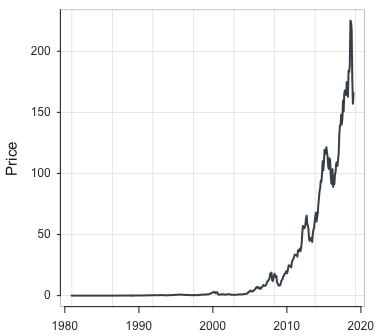## >>> Suggestions
## Plot(date, Price, fit="lm", fit_se=c(.90,.99))  # fit line, standard errors
## Plot(date, Price, out_cut=.10)  # label top 10% potential outliers
## Plot(date, Price, enhance=TRUE)  # many options
##
##
## >>> Pearson's product-moment correlation
##
## Number of paired values with neither missing, n = 458
##
##
## Sample Correlation of date and Price: r = 0.706
##
##
## Hypothesis Test of 0 Correlation:  t = 21.280,  df = 456,  p-value = 0.000
## 95% Confidence Interval for Correlation:  0.6570 to 0.7490

With the by parameter, plot all three companies on the same panel.

Plot(date, Price, by=Company)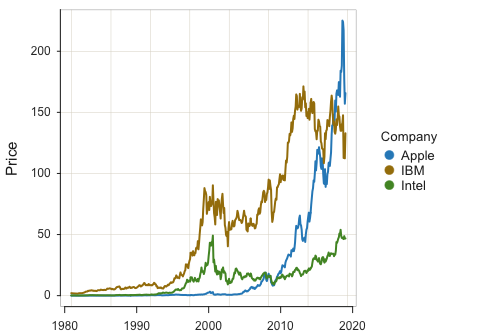## >>> Suggestions
## Plot(date, Price, fit="lm", fit_se=c(.90,.99))  # fit line, standard errors
## Plot(date, Price, out_cut=.10)  # label top 10% potential outliers
## Plot(date, Price, enhance=TRUE)  # many options
##
##
## >>> Pearson's product-moment correlation
##
## Number of paired values with neither missing, n = 1374
##
##
## Sample Correlation of date and Price: r = 0.677
##
##
## Hypothesis Test of 0 Correlation:  t = 34.036,  df = 1372,  p-value = 0.000
## 95% Confidence Interval for Correlation:  0.6470 to 0.7040

With the by1 parameter, plot all three companies on the different panels, a Trellis plot.

Plot(date, Price, by1=Company)
## [Trellis graphics from Deepayan Sarkar's lattice package]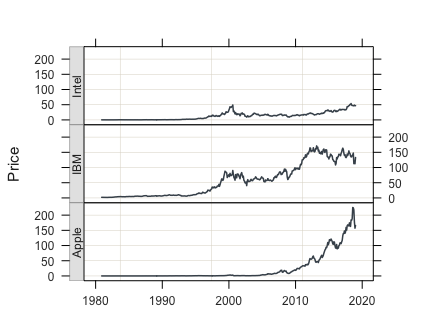Now do the Trellis plot with some color.

style(sub_theme="black", trans=.55,
window_fill="gray10", grid_color="gray25")
Plot(date, Price, by1=Company, n_col=1,  fill="darkred", color="red")
## [Trellis graphics from Deepayan Sarkar's lattice package]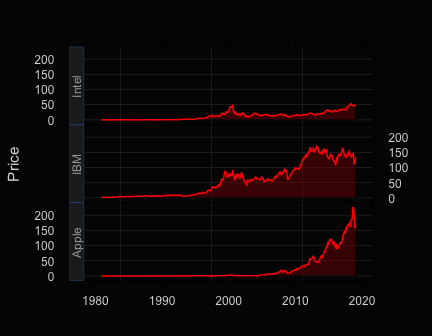With style() many themes can be selected, such as "lightbronze", "dodgerblue", "darkred", such as "gray". When no theme is specified, return to the default theme, colors.

style()
## theme set to "colors"

## Annotation

d <- Read("Employee")
##
## >>> Suggestions
## Details about your data, Enter:  details()  for d, or  details(name)
##
## Data Types
## ------------------------------------------------------------
## character: Non-numeric data values
## integer: Numeric data values, integers only
## double: Numeric data values with decimal digits
## ------------------------------------------------------------
##
##     Variable                  Missing  Unique
##         Name     Type  Values  Values  Values   First and last values
## ------------------------------------------------------------------------------------------
##  1     Years   integer     36       1      16   7  NA  15 ... 1  2  10
##  2    Gender character     37       0       2   M  M  M ... F  F  M
##  3      Dept character     36       1       5   ADMN  SALE  SALE ... MKTG  SALE  FINC
##  4    Salary    double     37       0      37   53788.26  94494.58 ... 56508.32  57562.36
##  5    JobSat character     35       2       3   med  low  low ... high  low  high
##  6      Plan   integer     37       0       3   1  1  3 ... 2  2  1
##  7       Pre   integer     37       0      27   82  62  96 ... 83  59  80
##  8      Post   integer     37       0      22   92  74  97 ... 90  71  87
## ------------------------------------------------------------------------------------------

Add three different text blocks at three different specified locations.

Plot(Years, Salary, add=c("Hi", "Bye", "Wow"), x1=c(12, 16, 18),
y1=c(80000, 100000, 60000))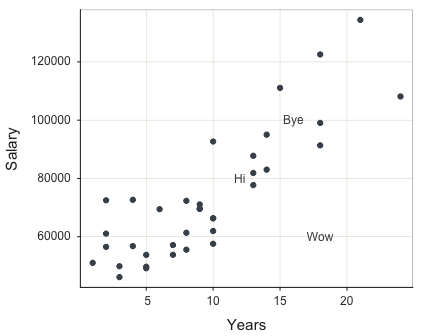## >>> Suggestions
## Plot(Years, Salary, fit="lm", fit_se=c(.90,.99))  # fit line, standard errors
## Plot(Years, Salary, out_cut=.10)  # label top 10% potential outliers
## Plot(Years, Salary, enhance=TRUE)  # many options
##
##
## >>> Pearson's product-moment correlation
##
## Number of paired values with neither missing, n = 36
##
##
## Sample Correlation of Years and Salary: r = 0.852
##
##
## Hypothesis Test of 0 Correlation:  t = 9.501,  df = 34,  p-value = 0.000
## 95% Confidence Interval for Correlation:  0.727 to 0.923

A rectangle requires two points, four coordinates, <x1,y1> and <x2,y2>.

style(add_trans=.8, add_fill="gold", add_color="gold4", add_lwd=0.5)
Plot(Years, Salary, add="rect", x1=12, y1=80000, x2=16, y2=115000)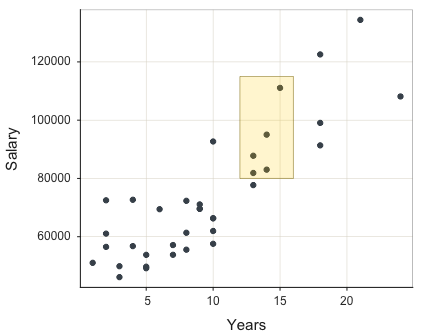## >>> Suggestions
## Plot(Years, Salary, fit="lm", fit_se=c(.90,.99))  # fit line, standard errors
## Plot(Years, Salary, out_cut=.10)  # label top 10% potential outliers
## Plot(Years, Salary, enhance=TRUE)  # many options
##
##
## >>> Pearson's product-moment correlation
##
## Number of paired values with neither missing, n = 36
##
##
## Sample Correlation of Years and Salary: r = 0.852
##
##
## Hypothesis Test of 0 Correlation:  t = 9.501,  df = 34,  p-value = 0.000
## 95% Confidence Interval for Correlation:  0.727 to 0.923

## Full Manual

Use the base R help() function to view the full manual for Plot(). Simply enter a question mark followed by the name of the function.

?Plot

## More

More on Scatterplots and other visualizations from lessR and other packages such as ggplot2 at:

Gerbing, D., R Visualizations: Derive Meaning from Data, CRC Press, May, 2020, ISBN 978-1138599635.